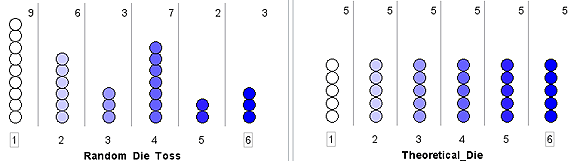Home > Statistics > Big ideas > Distribution > Empirical and theoretical

# Empirical and theoretical

The main distributions encountered at school are empirical distributions (created from data students collect) and theoretical distributions.

An example would be rolling a die many times and then comparing the results to a theoretical uniform distribution.

The figure below shows a comparison of 30 random tosses of a die and the theoretical uniform distribution of 5 of each outcome.Empirical and theoretical results for die tosses.

Different graphical representations show different aspects of distribution. By being exposed to many graph types during the middle years, students are led to ask the question: "How are my data distributed?"

Students can be led to consider skewness, symmetry, overall spread, outliers, means, medians, clumps and gaps.